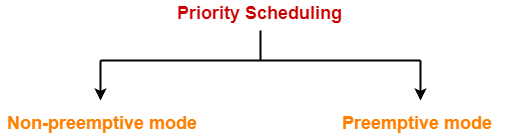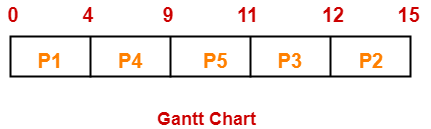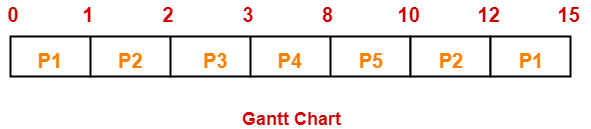# Priority Scheduling | CPU Scheduling | Examples

## Priority Scheduling-

In Priority Scheduling,

• Out of all the available processes, CPU is assigned to the process having the highest priority.
• In case of a tie, it is broken by FCFS Scheduling.• Priority Scheduling can be used in both preemptive and non-preemptive mode.

• It considers the priority of the processes and allows the important processes to run first.
• Priority scheduling in preemptive mode is best suited for real time operating system.

• Processes with lesser priority may starve for CPU.
• There is no idea of response time and waiting time.

## Important Notes-

### Note-01:

• The waiting time for the process having the highest priority will always be zero in preemptive mode.
• The waiting time for the process having the highest priority may not be zero in non-preemptive mode.

### Note-02:

Priority scheduling in preemptive and non-preemptive mode behaves exactly same under following conditions-

• The arrival time of all the processes is same
• All the processes become available

## Problem-01:

Consider the set of 5 processes whose arrival time and burst time are given below-

 Process Id Arrival time Burst time Priority P1 0 4 2 P2 1 3 3 P3 2 1 4 P4 3 5 5 P5 4 2 5

If the CPU scheduling policy is priority non-preemptive, calculate the average waiting time and average turn around time. (Higher number represents higher priority)

## Solution-

### Gantt Chart-Now, we know-

• Turn Around time = Exit time – Arrival time
• Waiting time = Turn Around time – Burst time

Also read- Various Times of Process

 Process Id Exit time Turn Around time Waiting time P1 4 4 – 0 = 4 4 – 4 = 0 P2 15 15 – 1 = 14 14 – 3 = 11 P3 12 12 – 2 = 10 10 – 1 = 9 P4 9 9 – 3 = 6 6 – 5 = 1 P5 11 11 – 4 = 7 7 – 2 = 5

Now,

• Average Turn Around time = (4 + 14 + 10 + 6 + 7) / 5 = 41 / 5 = 8.2 unit
• Average waiting time = (0 + 11 + 9 + 1 + 5) / 5 = 26 / 5 = 5.2 unit

## Problem-02:

Consider the set of 5 processes whose arrival time and burst time are given below-

 Process Id Arrival time Burst time Priority P1 0 4 2 P2 1 3 3 P3 2 1 4 P4 3 5 5 P5 4 2 5

If the CPU scheduling policy is priority preemptive, calculate the average waiting time and average turn around time. (Higher number represents higher priority)

## Solution-

### Gantt Chart-Now, we know-

• Turn Around time = Exit time – Arrival time
• Waiting time = Turn Around time – Burst time

 Process Id Exit time Turn Around time Waiting time P1 15 15 – 0 = 15 15 – 4 = 11 P2 12 12 – 1 = 11 11 – 3 = 8 P3 3 3 – 2 = 1 1 – 1 = 0 P4 8 8 – 3 = 5 5 – 5 = 0 P5 10 10 – 4 = 6 6 – 2 = 4

Now,

• Average Turn Around time = (15 + 11 + 1 + 5 + 6) / 5 = 38 / 5 = 7.6 unit
• Average waiting time = (11 + 8 + 0 + 0 + 4) / 5 = 23 / 5 = 4.6 unit

To gain better understanding about Priority Scheduling,

Watch this Video Lecture

Next Article- Practice Problems On CPU Scheduling Algorithms

Get more notes and other study material of Operating System.

Watch video lectures by visiting our YouTube channel LearnVidFun.

SummaryArticle Name
Priority Scheduling | CPU Scheduling | Examples
Description
Priority Scheduling is a CPU Scheduling Algorithm that assigns CPU to the process having the highest priority. Priority Scheduling Preemptive and Non-preemptive Examples.
Author
Publisher Name
Gate Vidyalay
Publisher Logo